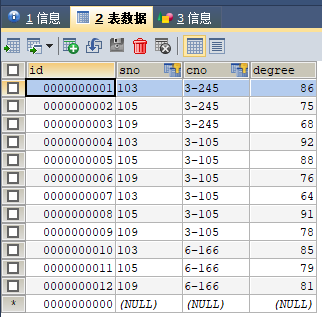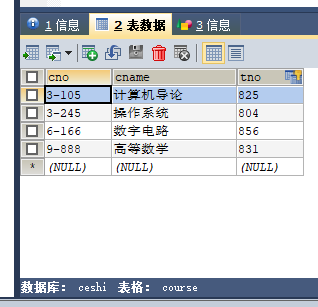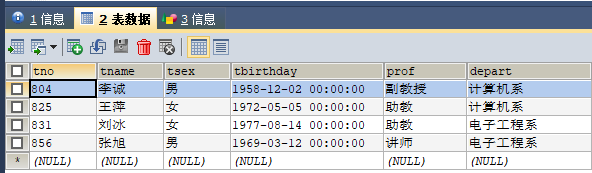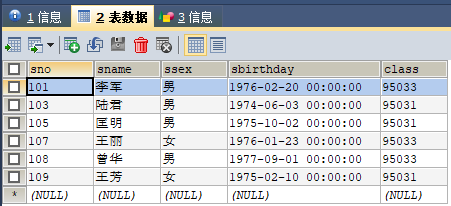## 数据库45道练习题 转

o
osc_agn9pfb01、 查询Student表中的所有记录的Sname、Ssex和Class列。

``SELECT sname,ssex,class FROM student;``

2、 查询教师所有的单位即不重复的Depart列。distinct

``SELECT DISTINCT depart FROM teacher;``

3、 查询Student表的所有记录。

``SELECT * FROM student;``

4、 查询Score表中成绩在60到80之间的所有记录。between and

``SELECT *FROM score WHERE degree BETWEEN 60 AND 80;``

5、查询Score表中成绩为85，86或88的记录

``````解法1：select * FROM score WHERE degree IN(85,86,88);

6、 查询Student表中“95031”班或性别为“女”的同学记录。

``select * FROM student WHERE class = '95031' OR ssex = '女';``

7、 以Class降序查询Student表的所有记录。order BY

``select * FROM student ORDER BY class DESC;``

8、 以Cno升序、Degree降序查询Score表的所有记录。

``select * FROM score ORDER BY cno,degree DESC;``

9、 查询“95031”班的学生人数。

``SELECT * FROM student WHERE class = '95031';``

10、查询Score表中的最高分的学生学号和课程号。（子查询或者排序）

``````SELECT sno,cno,degree FROM score WHERE degree = (SELECT MAX(degree) FROM score);子查询
SELECT sno,cno,degree FROM score ORDER BY degree DESC LIMIT 1;排列``````

11、查询每门课的平均成绩。

``SELECT cno,AVG(degree) FROM score GROUP BY cno;``

12、查询Score表中至少有5名学生选修的并以3开头的课程的平均分数。

``SELECT cno,AVG(degree) FROM score GROUP BY cno HAVING COUNT(*)>=5 AND cno LIKE '3%';``

13、查询分数大于70，小于90的Sno列。

``SELECT sno FROM score WHERE degree >70 AND degree <90;``

14、查询所有学生的Sname、Cno和Degree列。

``select aa.sname,bb.cno,bb.degree FROM student AS aa,score AS bb WHERE aa.`sno` = bb.`sno`;``

15、查询所有学生的Sno、Cname和Degree列。

``````解法1：
SELECT a.sname,b.degree,c.cname FROM student AS a,score AS b,course AS c WHERE a.sno = b.sno AND b.cno = c.cno;``````
``````解法2：
SELECT a.sname,b.degree,c.cname FROM student AS a JOIN score AS b ON a.sno = b.sno JOIN course AS c ON b.cno = c.cno;``````

16、 查询所有学生的Sname、Cname和Degree列。(三表联查)

``SELECT a.sno,b.degree,c.cname FROM student AS a JOIN score AS b ON a.sno = b.sno JOIN course AS c ON b.cno = c.cno;``

17、 查询“95031”班学生的平均分。

``SELECT AVG(degree) FROM score WHERE sno = ANY(SELECT sno FROM student WHERE class = '95031');``

create table grade(low  int(3),upp  int(3),rank  char(1))

``解法1：select sno,cno,rank from score,grade where degree between low and upp order by rank;``
``解法2：select sno,cno,degree,[rank]      from Score join  grade on degree between low and upp order by [rank]--on后面加筛选条件解法3：select Sno,Cno,(select [rank] from grade where Score.Degree between low and upp) as LV      from Score order by Degree desc--子查询得到的结果必须唯一``

19查询选修“3-105”课程的成绩高于“109”号同学成绩的所有同学的记录。

``select * from score where cno='3-105' and degree>(select degree from score  where sno=109 and cno='3-105');``

20、查询score中选学多门课程的同学中分数为非最高分成绩的记录。

``````理解1：选学多门课程的同学中，分数小于所有学生中最高分：
1.select * from score where sno in（2）and degree<（3）
2.select sno from score group by sno having count(*)>1
3.select max(degree) from score  where sno in（4）
4.select sno from score group by sno having count(*)>1

``````理解2：选多门课程中，分数小于每门课程最高分的学生信息
select * from score a where sno in(select sno from score group by sno having count(*)>1) anddegree<(select max(degree) from score  b where b.cno = a.cno )``````

21 查询成绩高于学号为“109”、课程号为“3-105”的成绩的所有记录。

``select *from score where degree>(select degree from score where sno='109'and  Cno='3-105')``

22、查询和学号为108的同学同年出生的所有学生的SnoSnameSbirthday

``select sno,sname,sbirthday from student where year(sbirthday)=(select year(sbirthday) from student where sno='108');``

23、查询张旭教师任课的学生成绩。

``````1.select * from score where cno in ()
2.select cno from course where tno=()
3.select tno from teacher where tname='张旭'

select * from score where cno in (select cno from course where tno=(select tno from teacher where tname='张旭'))``````
``解法1：select teacher.tname,course.cno,score.degree from teacher,course,score where teacher.tno=course.tno and course.cno=score.cno and tname='张旭';``
``解法2：select degree from Score join Course on Score.Cno=Course.Cno join Teacher on Course.Tno=Teacher.Tno where Tname = '张旭'``

24、查询选修某课程的同学人数多于5人的教师姓名。

``````1.select tname from teacher where tno=()
2.select tno from course where cno=()
3.select cno from score group by cno having count(*)>5

select tname from teacher where tno=(select tno from course where cno=(select cno from score group by cno having count(*)>5))``````
``解法1;select teacher.tname from teacher,score,course where teacher.tno=course.tno and course.cno=score.cno group by tname having count(*)>5 ;``
``解法2：select Tname from Teacher where Tno=(select Tno from Course where Cno=(select Cno from Score group by Cno having COUNT(Cno)>=5))``

25、查询95033班和95031班全体学生的记录。

``````解法1：select * from student where class='95033' or class='95031';

26、 查询存在有85分以上成绩的课程Cno.

``````select score.cno from score,course where score.cno=course.cno and degree>85;
select cno from score group by cno having max(degree)>85;``````

27、查询出计算机系教师所教课程的成绩表

``解法1：select degree from teacher,score,course where teacher.tno=course.tno and course.cno=score.cno and teacher.depart='计算机系';``
``解法2：select *from Score where Cno in( select Cno from Course where Tno in (select Tno from Teacher where Depart='计算机系'))解法3：select Sno,Score.Cno,Degree from Score join Course on Score.Cno=Course.Cno join Teacher on Course.Tno=Teacher.Tno where Depart='计算机系'``

28、查询计算 机系电子工程系不同职称的教师的TnameProf

``解法1：select tname,prof from teacher where depart='计算机系' and prof not in (select prof from teacher where depart='电子工程系');``
``````解法2：select tname,prof from teacher where prof not in
(select prof from teacher where depart='电子工程系' and prof in (select prof from teacher where depart='计算机系'))anddepart in ('计算机系','电子工程系')--查询两个系中教师相同职称名称，不在这里面的就是除去两个系都有的剩下的，见上面select prof from teacher where depart='电子工程系' and prof in (select prof from teacher where depart='计算机系')  ``````

29、查询选修编号为“3-105“课程且成绩至少高于选修编号为“3-245”的同学的CnoSnoDegree,并按Degree从高到低次序排序。any的用法

``解法1；select * from score where cno='3-105' and degree > (select min(degree) from score where cno='3-245')order by degree desc;``
``解法2：select * from score where cno='3-105' and degree > any(select degree from score where cno='3-245')order by degree desc;``

30、查询选修编号为“3-105”且成绩高于选修编号为“3-245”课程的同学的CnoSnoDegree.all的用法）

``解法1：select * from score where cno='3-105' and degree > (select max(degree) from score where cno='3-245');``
``解法2：select * from score where cno='3-105' and degree > all(select degree from score where cno='3-245');``

31 查询所有教师和同学的namesexbirthday.

``select tname,tsex,tbirthday from teacherunionselect sname,ssex,sbirthday from student``

32、查询所有教师和同学的namesexbirthday.

``select tname,tsex,tbirthday from teacher where tsex='女'unionselect sname,ssex,sbirthday from student where ssex='女'``

33 查询成绩比该课程平均成绩低的同学的成绩表。

``select * from score as aa where degree<(select avg(degree) from score as bb where aa.cno=bb.cno);``
``````解法2：详解--相关子查询--同一门学科的平均分,，每门学科低于自身平均分的select * from score as a where a.degree<
(select AVG(degree) from score as b where a.cno = b.cno  group by cno)--相当于foreach列出每一门课的平均分select * from score as a where a.degree<
(select AVG(degree) from score as b  group by cno having a.cno = b.cno)``````

34、 查询所有任课教师的TnameDepart

``解法1：select tname,depart from teacher where exists (select * from course where teacher.tno=course.tno);``
``解法2：select Tname,Depart from Teacher where Tno in(select distinct Tno from Course)``

35 、 查询所有未讲课的教师的TnameDepart.

``解法1：select tname,depart from teacher where not exists (select * from course where teacher.tno=course.tno);``
``解法2：select Tname,Depart from Teacher where Tno in     (select Tno from Course where Cno not in(select Cno from Score group by Cno))解法3：select Tname,Depart from Teacher where Tno not in(select Tno from Course)``

36、查询至少有2名男生的班号。

``select class from student where ssex='男'group by class having count(*)>=2;``

37、查询Student表中不姓的同学记录。

``select * from student where sname not like'王%';``

38、查询Student表中每个学生的姓名和年龄。

``select sname,year(now())-year(sbirthday) from student;``

39、查询Student表中最大和最小的Sbirthday日期值。

``select max( date(student.sbirthday)) ,min( date(student.sbirthday)) from student;``

40、以班号和年龄从大到小的顺序查询Student表中的全部记录。

``select * from student order by class desc,date(sbirthday) asc;``

41、查询教师及其所上的课程。

``解法1：select teacher.tname,teacher.tsex,course.cname from teacher,course where teacher.tno=course.tno and tsex='男';``
``解法2：select teacher.tno,tname,tsex,cname,cno from teacher join course on teacher.tno=course.tno where tsex='男'``

42、查询最高分同学的SnoCnoDegree列。

``select sno,cno,degree from score where degree=(select max(degree) from score);``

43、查询和李军同性别的所有同学的Sname.

``select sname from student where ssex=(select ssex from student where sname='李军');``

44、查询和李军同性别并同班的同学Sname.

``select sname from student where ssex=(select ssex from student where sname='李军') and class=(select class from student where sname='李军');``

45、查询所有选修计算机导论课程的同学的成绩表。

``````select student.sname,score.degree from student,score,course where student.sno=score.sno and course.cno=score.cno and course.cname='计算机导论' and student.ssex='男';
select * from score where sno in(select sno from student where ssex='男') and cno=(select cno from course where cname='计算机导论')``````
o

### osc_agn9pfb0

2013/10/27
1.7K
7
【opencv】图形的绘制

1.矩形图像的绘制： 原函数：void cvRectangle(CvArr* img, CvPoint pt1, CvPoint pt2, CvScalar color, int thickness=1, int line_type=8,int shift=0) img就是需要绘制的图像 pt1 and pt......

2014/10/08
1.2K
1

MaoCaiJun.DataBase 是一个用于 Microsoft Visual Studio 的数据库代码生成组件。它是基于 xml 文件的代码创建工具，支持sql2000,sql2005,sql2008,access, SQLite MaoCaiJun.Database 数据库...

mccj
2013/02/06
2.3K
1

SQLScreens 是一个使用 Tcl/TK 编写的简单关系型数据库表单生成工具。可让你快速创建查询界面，并指定相应的表和字段。支持多种数据库，包括：MySQL, SQLite, and INFORMIX, and ODBC for o...

2013/02/17
910
0

edbc 是 Emacs-Lisp Database Connectivity 的缩 写 ，是从 Emacs-CGI 项目中独立出来的一个子项目，为 Emacs Lisp 访问数据库提供简洁、统一的接口！ 在其他语言（比如Java）中访问数据库，...

redraiment
2012/12/26
1.6K
0

PPDet：减少Anchor-free目标检测中的标签噪声，小目标检测提升明显

0
0
BIO、NIO、AIO 区别和应用场景

0
0
ContentProvider（查询 插入 修改 删除 ）

osc_6ttvlt1w
43分钟前
30
0

11
0

osc_wdq5dwoy
45分钟前
0
0